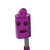# The sum of three consecutive multiples of 12 is 468. Find these multiples .

The sum of three consecutive multiples of 12 is 468. Find these multiples .

### 2 thoughts on “The sum of three consecutive multiples of 12 is 468. Find these multiples .”

1.let the three multiples be 12x, 12x+12, and 12x+24.

from given,

(12x) + (12x+12) + (12x+24) = 468

36x + 36 = 468

36x = 468 – 36 = 432

x = 432/36

x = 12

the three multiples are 12x = 12(12) = 144

12x + 12 = 12(12) + 12 = 156

12x + 24 = 12(12) + 24 = 168

Hope this helps!!

Pls mark me as brainliest!!

2.Step-by-step explanation:

Let the multiples be x,x+12 ,x +24

x+x+12+x+24 = 468

3x + 36 = 468

3x = 468-36

X = 432/3

X= 144

so the no’s are

x = 144

x+12 =156

x+24=168